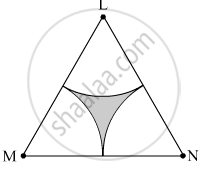# Δ Lmn is an Equilateral Triangle. Lm = 14 Cm. as Shown in Figure, Three Sectors Are Drawn with Vertices as Centres and Radius 7 Cm. Find, Total Area of All the Three Sectors. - Geometry

#### Question

$∆$ LMN is an equilateral triangle. LM = 14 cm. As shown in the figure, three sectors are drawn with vertices as centers and radius 7 cm.
Find, Total area of all the three sectors.#### Solution

∆LMN is an equilateral triangle.
∴ LM = MN = LN = 14 cm
∠L = ∠M = ∠N = 90º

Total area of all the three sectors = 3 × Area of any one of the sectors = 3 × 25.67 = 77.01 cm2

Is there an error in this question or solution?